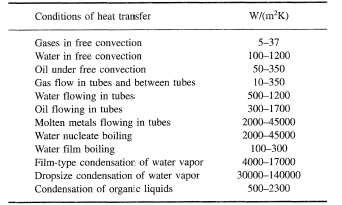Overall Heat Transfer Coefficient

Overall Heat Transfer Coefficient Thermodynamics

Related Resources:

In the case of combined heat transfer, it is common practice to relate the total rate of heat transfer (Q), the overall cross-sectional area for heat transfer (Ao), and the overall temperature difference (ΔTo) using the overall heat transfer coefficient (Uo). The overall heat transfer coefficient combines the heat transfer coefficient of the two heat exchanger fluids and the thermal conductivity of the heat exchanger tubes. Uo is specific to the heat exchanger and the fluids that are used in the heat exchanger.˙

Q = UoAo ΔTo

where: ˙

Q = the rate heat of transfer (Btu/hr)
Uo= the overall heat transfer coefficient (Btu/hr -ft2 -oF)
Ao= the overall cross-sectional area for heat transfer (ft2)
ΔTo= the overall temperature difference (oF)Membership Register | LoginHomeEngineering Book StoreEngineering ForumExcel App. DownloadsOnline Books & ManualsEngineering NewsEngineering VideosEngineering CalculatorsEngineering ToolboxGD&T Training Geometric Dimensioning TolerancingDFM DFA TrainingTraining Online EngineeringAdvertising CenterCopyright Notice